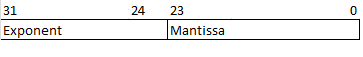# GATE | GATE CS 1996 | Question 25

Consider the following floating point number representationThe exponent is in 2’s complement representation and mantissa is in the sign magnitude representation. The range of the magnitude of the normalized numbers in this representation is
a). 0 to 1
b). 0.5 to 1
c). 2-23 to 0.5
d). 0.5 to (1-2-23)
(A) a
(B) b
(C) c
(D) d

Explanation: Normalized numbers are of the form: 1.xxxx xxxx… …xxxx {ignoring sign and exponent part}. They always have a Leading 1 before decimal point.
While storing such a number in this floating point representation two cases can be there:

• Case 1: We can store most significant 1 in mantissa along with other bits as (while MSB of mantissa is a sign bit as ‘S’) :
Minimum Mantissa=> S100 0000 0000 0000 0000 0000 = 1.00 0000…0000 = 1
Maximum Mantissa=> S111 1111 1111 1111 1111 1111 = 1.1111…1111 = 2 – 2-22
• Case 2: We can ignore to do so assuming an implicit MSB as 1.
Minimum Mantissa=> S000 0000 0000 0000 0000 0000 = 1.000 0000…0000 = 1
Maximum Mantissa=> S111 1111 1111 1111 1111 1111 = 1.1111…1111 = 2 – 2-23

Thus none of the options matches.

If instead of Normalized numbers they had asked for Normalized mantissa then following would have been the approach:
minimum value of magnitude of Mantissa=>
“S100 0000 0000 0000 0000 0000”
(ignoring (-1)s as a multiple of the number as only magnitude has been asked)

```= 0.100 0000 0000 0000 0000 0000
= 1*2-1
= 0.5
and
maximum value of Mantissa=>
“S111 1111 1111 1111 1111 1111”
= 0.111 1111 1111 1111 1111 1111
= [(223)- 1] * 2-23
= 1-2-23 ```

In this case option (D) would be the answer.

This explanation is contributed by Pradeep Pandey.

Quiz of this Question

My Personal Notes arrow_drop_up